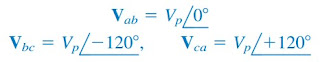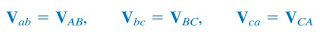omG8HI9LSKFW6lhCj8prTs0Z6lUhdOT9Jhi1Sf4m

# Three-Phase Electric Circuit: Balanced Delta-Delta Connection

A delta-delta system is a system where the source is delta-connected where the load is also delta-connected.
A balanced Delta-Delta system is one in which both the balanced source and balanced load are ∆-connected.
Before moving on make sure you have read what is three-phase circuit first.

## Balanced Delta-Delta Connection

The source, as well as the load, may be delta-connected as shown in Figure.(1). Our goal is to obtain the phase and line currents as usual.Figure 1. A balanced ∆- ∆ connection
Assuming a positive sequence, the phase voltages for a delta-connected source are(1)
The line voltages are the same as the phase voltages. From Figure.(1), assuming there are no line impedances, the phase voltages of the delta-connected source are equal to the voltages across the impedances; that is,(2)
Hence, the phase currents are(3)
Since the load is delta-connected just as in the balanced wye-delta connection, some of the formulas derived there apply here. The line currents are obtained from the phase currents by applying KCL at nodes A, B, and C, as we did in the previous section:(4)
Also, as shown in the last section, each line current lags the corresponding phase current by 30◦; the magnitude IL of the line current is √3 times the magnitude Ip of the phase current,(5)
An alternative way of analyzing the ∆-∆ circuit is to convert both the source and the load to their Y equivalents. We already know that ZY = Z∆/3. To convert a ∆-connected source to a Y-connected source, see the next section.

## Balanced Delta-Delta Connection Example

For better understanding let us review the example below:
1. A balanced ∆-connected load having an impedance 20 − j15 Ω is connected to a ∆-connected, positive-sequence generator having Vab = 330∠0◦ V. Calculate the phase currents of the load and the line currents.
Solution:
The load impedance per phase is
The phase currents are
For a delta load, the line current always lags the corresponding phase current by 30◦ and has a magnitude √3 times that of the phase current.
Hence, the line currents are

Have you understood what is balanced delta-delta connection? Don't forget to share and subscribe! Happy learning!
Reference:  Fundamentals of electric circuits by Charles K. Alexander and Matthew N. O. Sadiku
Related Posts
SHARE